# GMAT Math : Calculating the perimeter of a right triangle

## Example Questions

### Example Question #1 : Calculating The Perimeter Of A Right Triangle

What is the perimeter of a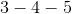traingle?Explanation:

Atriangle is a right triangle. To find the perimeter, we must add up all the sides of the triangle.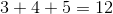### Example Question #2 : Calculating The Perimeter Of A Right Triangle

A right triangle has a hypotenuse of length 13 yards and a leg of length 5 yards. Give the perimeter of this triangle in inches.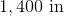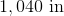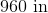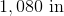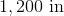Explanation:

We can use the Pythagorean Theorem to calculate the length of the second legin yards of the triangle by setting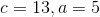in this formula: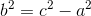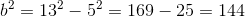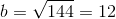The perimeter of the triangle is yards is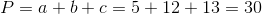yards. Multiply this by 36 to covert to inches: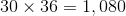inches.

### Example Question #3 : Calculating The Perimeter Of A Right Triangle

A right triangle has legs of lengthfeet and and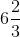feet. Give the perimeter of this triangle in yards.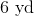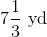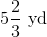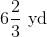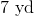Explanation:

We can use the Pythagorean Theorem to calculate the hypotenuseof the triangle by setting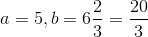in this formula: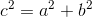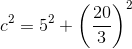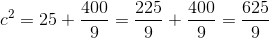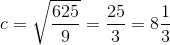The perimeter is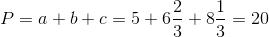feet.

Divide by 3 to convert to yards: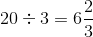yards

### Example Question #4 : Calculating The Perimeter Of A Right Triangle

What is the perimeter of a 30-60-90 triangle?

1) One of the sides measures 10 inches.

2) One of the sides measures 20 inches.

EACH statement ALONE is sufficient.

Statement 1 ALONE is sufficient, but Statement 2 alone is not sufficient.

Statements 1 and 2 TOGETHER are not sufficient.

BOTH statements TOGETHER are sufficient, but neither statement ALONE is sufficient.

Statement 2 ALONE is sufficient, but Statement 1 alone is not sufficient.

BOTH statements TOGETHER are sufficient, but neither statement ALONE is sufficient.

Explanation:

The hypotenuse of a 30-60-90 triangle measures twice its shorter leg; its longer leg measures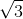times its shorter leg. Given one side length alone, there is no indication which of the three sides it measures; but given two, one of which is twice the other, as is the case here (10 and 20), 10 must be the shorter leg and 20 the hypotenuse. The longer leg is therefore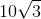, and the perimeter is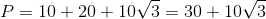.

Therefore, both statements together are sufficent but neither alone is sufficient.

### Example Question #5 : Calculating The Perimeter Of A Right Triangle

A right triangle has a base of 4 and a height of 3. What is the perimeter of the triangle?Explanation:

We are given two sides of the right triangle, so in order to calculate the perimeter we must first find the length of the third side, the hypotenuse, using the Pythagorean theorem: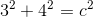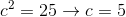Now that we know the length of the third side, we can add the lengths of the three sides to calculate the perimeter of the right triangle: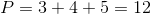### Example Question #6 : Calculating The Perimeter Of A Right Triangle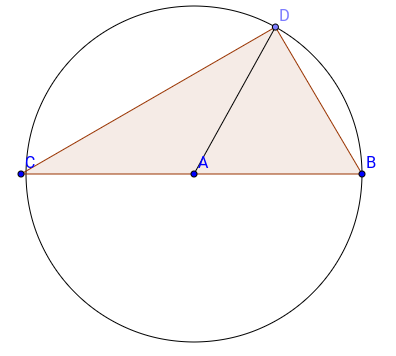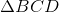is a right triangle and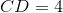and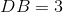. What is half the circle's circumference added to the triangle's perimeter?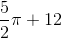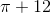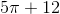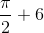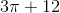As you see that a right triangle as sides 3 and 4, you should always remember that, this right triangle is a Pythagorean Triple, in other words, its sides will be in the ratio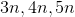whereis a constant, this will save you a lot of time. Here we can say that the hypotenuse will be 5. Therefore, the circumference will be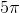. To get the final answer, we should just divide the circumference by 2 and add the perimeter, being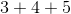or 12.